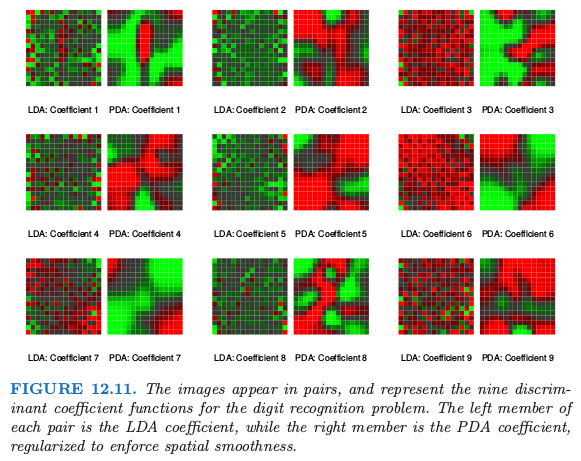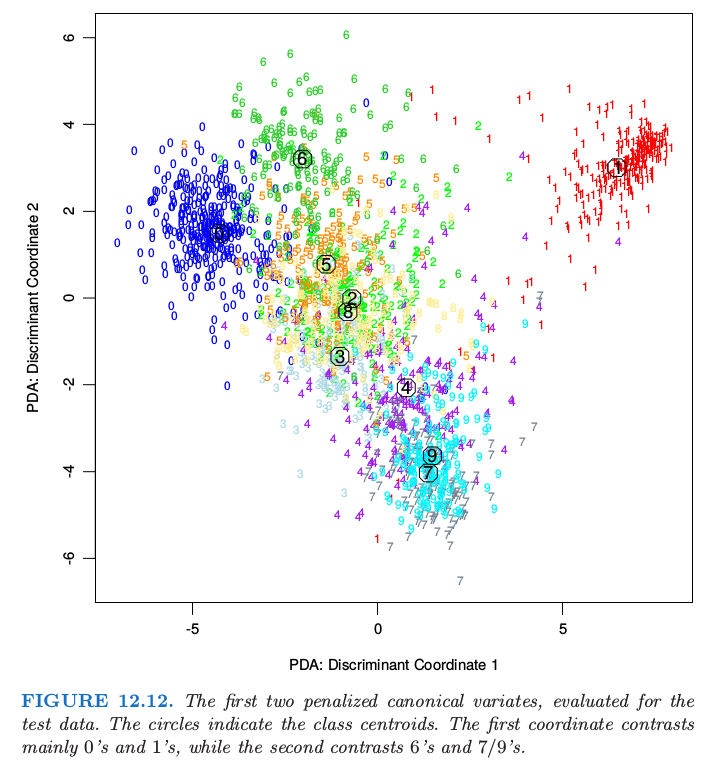12.6 惩罚判别分析¶

$\mathbf \Omega$ 的选择取决于具体问题．如果 $\eta_\ell(x)=h(x)\beta_\ell$ 是样条基函数的展开，则 $\mathbf\Omega$ 可能约束 $\eta_\ell$ 要在 $\IR^p$ 上光滑．在可加样条的情形下，每个坐标有 $N$ 个样条基函数，则 $h(x)$ 总共包含 $Np$ 个基函数；在这种情形下，$\mathbf\Omega$ 是 $Np\times Np$ 的，并且是分块对角的．

• 通过基展开 $h(X)$ 扩充预测变量 $X$ 的集合；
• 在增广空间中采用（带惩罚的）LDA，其中带惩罚的 马氏距离 (Mahalanobis distance) 由下式给出 其中 $\mathbf \Sigma_W$ 是导出变量 $h(x_i)$ 的类别内协方差矩阵．
• 采用惩罚度量分解分类子空间： 不严谨地说，带惩罚的麦哈顿距离趋向于给“粗糙”的坐标更少的权重，而给“光滑”的坐标更多的权重；因为惩罚不是对角的，同样的方式会应用到粗糙或光滑的线性组合中．

• 语音片段的对数周期图，在 256 个频率的集合上进行采样；见图 5.5．
• 手写数字的数字图像中的灰度像素值．